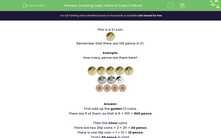# Counting Cash: Value of Coins in Pence

In this worksheet, students must recognise the given coins and work out the total value in pence.Key stage:  KS 1

Curriculum topic:   Measurement

Curriculum subtopic:   Use Combinations of Coins

Difficulty level:#### Worksheet Overview

This is a £1 coin.Remember that there are 100 pence in £1.

Example:

How many pence are there here?First add up the golden £1 coins.

There are 5 of them, so that is 5 × 100 = 500 pence.

Then the silver coins.

There are two 20p coins = 2 × 20 = 40 pence.

There is one 10p coin = 1 × 10 = 10 pence.

That's 50 pence in total.

Then the bronze coins.

There are four 1p coins = 4 × 1 = 4 pence.

In total, that is 500 + 50 + 4 = 554 pence or £5 and 54 pence.

### What is EdPlace?

We're your National Curriculum aligned online education content provider helping each child succeed in English, maths and science from year 1 to GCSE. With an EdPlace account you’ll be able to track and measure progress, helping each child achieve their best. We build confidence and attainment by personalising each child’s learning at a level that suits them.

Get started